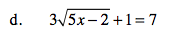### Home > A2C > Chapter 5 > Lesson 5.1.2 > Problem5-27

5-27.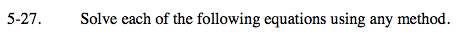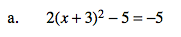2(x + 3)2 = 0

(x + 3)2 = 0

x = −3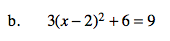See part (a).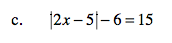$\mid 2\textit{x} - 5 \mid = 21$

2x − 5 = ± 21

x = −8 or x = 13

$\sqrt{5\textit{x}-2} = 2$